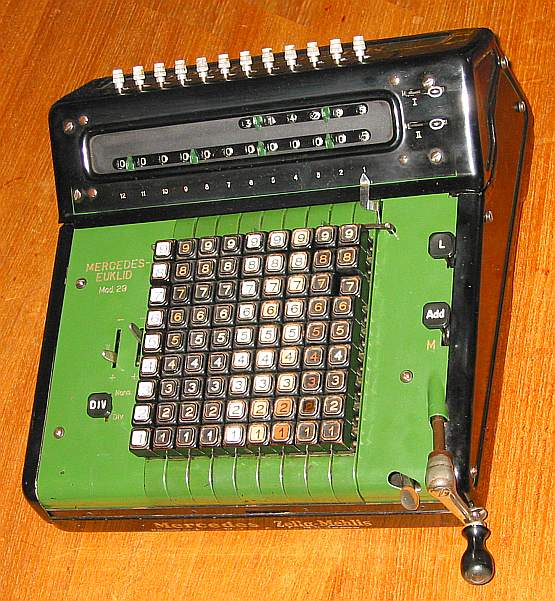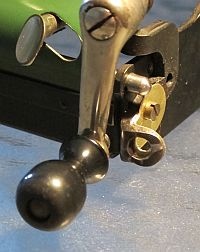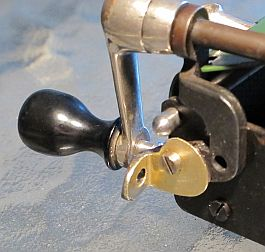previous <<==>> next

MERCEDES-EUKLID 29```The picture shows the status after the division 22:7 = 3.14287 rem. = 5 T E C H N I C A L D A T A of the MERCEDES-EUKLID Model » 29 « ************************************************************************ Invented by CHRISTEL HAMANN in 1906 Serial-Number: 36698 Dimensions: (ca.) Width = 12_1/2" / 32 cm Depth = 15 " / 38 cm Height = 7 " / 18 cm Weight: (ca.) 26_1/2 lbs / 12 kg Mechanics: 9*9 Full-Keyboard Proportional-Lever / Proportional-Hebel Functions: Add, Subtract, Multiply, Divide AUTOMATED DIVISION ! Registers: I Counter = 6 Decimals II Arithmetic = 12 Decimals III Input = 9 Decimals Manufacturer: MERCEDES BUEROMASCHINEN WERKE Zella-Mehlis in Thueringen Germany 1935 BASICS & HOW TO USE the MERCEDES-EUKLID 29 ********************************************** (A) Clearing Registers: ----------------------- The upper right levers clear the register I = Counter & II = Arithmetic. Register III = Input = Keyboard will be cleared with Key [L]. Key [Add] has two positions: Pushed down & foreward to (A = Addition) the keyboard will be cleared after operation - Pulled up to (M= Multiplication) the keyboard will NOT be cleared after operation ( = "Repeat" Function ). (B) Shifting the Carriage: -------------------------- The carriage has to be shifted to the right by hand. Shift to the left is performed step-by-step with the SILVER LEVER left beside the crank. (C) Carriage Settings & Counting Directions: -------------------------------------------- The arithmetic register (II) is PRE-SETable with 12 metal knobs on top of the carriage. Lever I ( left beside the keyboard ) controls the counter (I) and has 2 positions: When set to (+), register (I) counts "positive"; When set to (-) register (I) counts "negative". "Positive" means the counter & arithmetic unit are working in the same; in case of "negative", both are working in opposite direction. Prior to each task registers (I), (II) & (III) have to be cleared; Default settings: Carriage in the leftmost position, Key [Add] to (A), Levers I & II to (+), Key [DIV] pulled-up to (Norm). ADDITION & SUBTRACTION: ======================= Example: 123 + 45 - 6 = 162 ADD: Enter the first number (123) in the far right columns. Pull the handle and turn the crank ( clockwise only! ) to transfer the number into arithmetic unit. Let the crank snap into the lock. The counting unit displays the figure 1. Enter the second number (45). Turn the crank to add the number. The arithmetic unit displays the intermediate sum (168) and the counting unit displays the figure 2. SUBTRACT: Enter the third number (6). Switch Lever II to (-). Turn the crank ( clockwise only! ) to subtract the number. The arithmetic unit displays the result (162) and the counting unit displays the figure 3. REMARK: NEGATIVE RESULTS are displayed in the arithmetic unit as the COMPLEMENT of the next higher 10, 100, 1000, ... Example: -12 = 99...9988 MULTIPLICATION: =============== Example: 123 * 45 = 5535 Clear I & II & III, pull Key [Add] to (M), Levers I & II to (+). Enter the multiplicand (123) in the far right columns of the keyboard. The multiplicator (45) has two digits, so the arithmetic unit has to be moved to position 2 by hand. Turn the crank until the first figure of the multiplicator (4) will appear in the second position of the counter. Move the arithmetic unit with the silver lever to position 1. Turn the crank until the second figure of the multiplicator (5) will appear in the first position of the counter. The multiplication is done: The multiplicand (123) stays in the keyboard, the multiplicator (45) is in the counter unit and the result (5535) is in the arithmetic unit. DIVISION: ========= Example: 22 : 7 = 3.14285 Remainder 5 Clear Registers I & II & III, pull Key [Add] to (M), push Key [DIV] to (Div) ... this forces Levers I to (-) & II to (+) Division requires 3 steps: (A) Set the Dividend into Arithmetic Unit: -------------------------------------- For the maximum number of decimals, shift the arithmetic unit to the far right position by hand. PRE-SET the arithmetic unit with the divident (22) in position 7.. (B) Set the Divisor in the Keyboard: -------------------------------- Enter the divisor (07) right into keyboard below the dividend (22). (C) Divide: ------- Turn the crank until it stops! The division procedure is automated: Subtractions until "Underflow", Addition, Carriage, Subtractions ... The result (3.14285) is in the counter (I) unit, and the remainder (5) is in the arithmetic (II) unit. The divisor (7) stays in the keyboard, therefore an additional decimal can be estimated ... ( 7, cause 7 x 7 = 49 ). Have a look at "Calculating Trickies" ... HOW TO DISASSEMBLE MODEL 29 FOR MAINTENANCE ACCESS ... R E M A R K S T O R E S T O R A T I O N : ======================================================================== Sorry, the DELETE-CAPS are missing (replaced by insulator pipes) The CRANK-REST was broken during transport and had to be restored: Using a brass sheet support, holding the "Horse-Shoe" with 2-Component-Glue...impressum: ************************************************************************ © C.HAMANN http://public.BHT-Berlin.de/hamann 06/30/11 ```